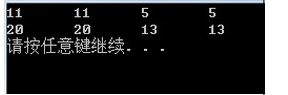# 如何求C语言字符串长度（strlen函数和sizeof关键字）

----------------------------------------------------------------------------------------------------------------------------------------------------------

C语言字符串长度的计算是编程时常用到的，也是求职时必考的一项。

C语言本身不限制字符串的长度，因而程序必须扫描完整个字符串后才能确定字符串的长度。

void test6()
{
char s1 = "hello world";
char s2 = {'h','e','l','l','0',' ','w','o','r','l','d','\0'};
char s3[] = "hello\0 world";
char s4[] = {'h','e','l','l','0','\0',' ','w','o','r','l','d','\0'};
printf("%d\t%d\t%d\t%d\n",strlen(s1),strlen(s2),strlen(s3),strlen(s4));
printf("%d\t%d\t%d\t%d\n",sizeof(s1),sizeof(s2),sizeof(s3),sizeof(s4));
}1. strlen函数求得的字符串长度是从字符串第一个元素到第一个'\0'之间元素的个数(如果字符串中间有'\0',则结果不是整个字符串的长度)，同时不包括该'\0'

2. sizeof求得的结果是存储该字符串的变量占用的空间大小，因而一定会包括'\0'.若'\0'后还有空余的空间，也会包含到结果里面

1. strlen()的一种实现就是遍历字符串，遇到'\0'就终止，因而返回的结果是第一个'\0'前字符元素的个数

2. sizeof 常用来求变量占用内存空间的大小，因而它返回的是存储字符串的变量所占用的内存空间大小，用来求字符串的长度，只在特定情况下可行，即字符数组刚好被一个字符串占满。

---------------------------------------------------------------------------------------------------------------------------------------------------------

char a1[] = "abc";
int a2;
sizeof( a1 ); // 结果为4，字符末尾还存在一个NULL终止符
sizeof( a2 ); // 结果为3*4=12（依赖于int）

int c1=sizeof(a1)/sizeof(char);//总长度/单个元素的长度  char型
int c2=sizeof(a2)/sizeof(a2);//总长度/第一个元素的长度 int型